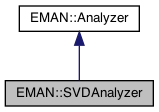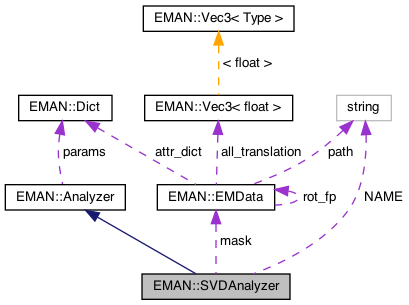EMAN2
EMAN::SVDAnalyzer Class Reference

Singular Value Decomposition from GSL. More...

`#include <analyzer.h>`

Inheritance diagram for EMAN::SVDAnalyzer:[legend]
Collaboration diagram for EMAN::SVDAnalyzer:[legend]

## Public Member Functions

SVDAnalyzer ()

virtual int insert_image (EMData *image)
insert a image to the list of input images More...

virtual int insert_images_list (vector< EMData * > image_list)
insert a list of images to the list of input images More...

virtual vector< EMData * > analyze ()
main function for Analyzer, analyze input images and create output images More...

string get_name () const
Get the Analyzer's name. More...

string get_desc () const
Get the Analyzer's description. More...

void set_params (const Dict &new_params)
Set the Analyzer parameters using a key/value dictionary. More...

TypeDict get_param_types () const
Get Analyzer parameter information in a dictionary. More...Public Member Functions inherited from EMAN::Analyzer
Analyzer ()

virtual ~Analyzer ()

virtual Dict get_params () const
Get the Reconstructor's parameters in a key/value dictionary. More...

## Static Public Member Functions

static AnalyzerNEW ()

## Static Public Attributes

static const string NAME = "svd_gsl"

## Protected Attributes

int nvec

int pixels

int nimgProtected Attributes inherited from EMAN::Analyzer
Dict params

vector< EMData * > images

int nsofar

gsl_matrix * A

## Detailed Description

Singular Value Decomposition from GSL.

Comparable to pca

Parameters
 mask mask image nvec number of desired basis vectors nimg total number of input images, required even with insert_image()

Definition at line 307 of file analyzer.h.

## ◆ SVDAnalyzer()

 EMAN::SVDAnalyzer::SVDAnalyzer ( )
inline

Definition at line 310 of file analyzer.h.

310: mask(0), nvec(0), nimg(0), A(NULL) {}
gsl_matrix * A
Definition: analyzer.h:360
Definition: analyzer.h:353

Referenced by NEW().

## ◆ analyze()

 vector< EMData * > SVDAnalyzer::analyze ( )
virtual

main function for Analyzer, analyze input images and create output images

Returns
vector<EMData *> result os images analysis

Implements EMAN::Analyzer.

Definition at line 959 of file analyzer.cpp.

960{
961// Allocate the working space
962gsl_vector *work=gsl_vector_alloc(nimg);
963gsl_vector *S=gsl_vector_alloc(nimg);
964gsl_matrix *V=gsl_matrix_alloc(nimg,nimg);
965gsl_matrix *X=gsl_matrix_alloc(nimg,nimg);
966
967
968// Do the decomposition. All the real work is here
969gsl_linalg_SV_decomp_mod (A,X, V, S, work);
970//else gsl_linalg_SV_decomp_jacobi(A,V,S);
971
972vector<EMData*> ret;
973//unpack the results and write the output file
976for (int k=0; k<nvec; k++) {
977 EMData *img = new EMData;
979
980 float *d=img->get_data();
981 for (size_t i=0,j=0; i<totpix; ++i) {
982 if (md[i]) {
983 d[i]=(float)gsl_matrix_get(A,j,k);
984 j++;
985 }
986 }
987 img->set_attr( "eigval", gsl_vector_get(S,k));
988 ret.push_back(img);
989}
990
991gsl_vector_free(work);
992gsl_vector_free(S);
993gsl_matrix_free(V);
994gsl_matrix_free(X);
995
996gsl_matrix_free(A);
997A=NULL;
999
1000return ret;
1001}
#define V(i, j)
Definition: analyzer.cpp:697
EMData stores an image's data and defines core image processing routines.
Definition: emdata.h:82

References A, mask, nimg, nvec, and V.

## ◆ get_desc()

 string EMAN::SVDAnalyzer::get_desc ( ) const
inlinevirtual

Get the Analyzer's description.

Returns
The Analyzer's description.

Implements EMAN::Analyzer.

Definition at line 329 of file analyzer.h.

330 {
331 return "Singular Value Decomposition from GSL. Comparable to pca";
332 }

## ◆ get_name()

 string EMAN::SVDAnalyzer::get_name ( ) const
inlinevirtual

Get the Analyzer's name.

Each Analyzer is identified by a unique name.

Returns
The Analyzer's name.

Implements EMAN::Analyzer.

Definition at line 324 of file analyzer.h.

325 {
326 return NAME;
327 }
static const string NAME
Definition: analyzer.h:350

References NAME.

## ◆ get_param_types()

 TypeDict EMAN::SVDAnalyzer::get_param_types ( ) const
inlinevirtual

Get Analyzer parameter information in a dictionary.

Each parameter has one record in the dictionary. Each record contains its name, data-type, and description.

Returns
A dictionary containing the parameter info.

Implements EMAN::Analyzer.

Definition at line 341 of file analyzer.h.

342 {
343 TypeDict d;
345 d.put("nvec", EMObject::INT, "number of desired basis vectors");
346 d.put("nimg", EMObject::INT, "total number of input images, required even with insert_image()");
347 return d;
348 }

References EMAN::EMObject::EMDATA, EMAN::EMObject::INT, and EMAN::TypeDict::put().

## ◆ insert_image()

 int SVDAnalyzer::insert_image ( EMData * image )
virtual

insert a image to the list of input images

Parameters
 image
Returns
int 0 for success, <0 for fail

Implements EMAN::Analyzer.

Definition at line 937 of file analyzer.cpp.

938{
940 throw NullPointerException("Null mask image pointer, set_params() first");
941
942 // count pixels under mask
944 float *d=image->get_data();
946 for (size_t i=0,j=0; i<totpix; ++i) {
947 if (md[i]) {
948 gsl_matrix_set(A,j,nsofar,d[i]);
949 j++;
950 }
951 }
952 nsofar++;
953
954 return 0;
955}
#define NullPointerException(desc)
Definition: exception.h:241

References A, mask, nsofar, and NullPointerException.

## ◆ insert_images_list()

 virtual int EMAN::SVDAnalyzer::insert_images_list ( vector< EMData * > image_list )
inlinevirtual

insert a list of images to the list of input images

Parameters
 image_list
Returns
int 0 for success, <0 for fail

Reimplemented from EMAN::Analyzer.

Definition at line 314 of file analyzer.h.

314 {
315 vector<EMData*>::const_iterator iter;
316 for(iter=image_list.begin(); iter!=image_list.end(); ++iter) {
317 images.push_back(*iter);
318 }
319 return 0;
320 }
vector< EMData * > images
Definition: analyzer.h:117

References EMAN::Analyzer::images.

## ◆ NEW()

 static Analyzer * EMAN::SVDAnalyzer::NEW ( )
inlinestatic

Definition at line 334 of file analyzer.h.

335 {
336 return new SVDAnalyzer();
337 }

References SVDAnalyzer().

## ◆ set_params()

 void SVDAnalyzer::set_params ( const Dict & new_params )
virtual

Set the Analyzer parameters using a key/value dictionary.

Parameters
 new_params A dictionary containing the new parameters.

Reimplemented from EMAN::Analyzer.

Definition at line 1003 of file analyzer.cpp.

1004{
1005 params = new_params;
1007 nvec = params["nvec"];
1008 nimg = params["nimg"];
1009
1010 // count pixels under mask
1011 pixels=0;
1014 for (size_t i=0; i<totpix; ++i) if (d[i]) ++pixels;
1015
1016 printf("%d,%d\n",pixels,nimg);
1017 A=gsl_matrix_alloc(pixels,nimg);
1018 nsofar=0;
1019}

References A, mask, nimg, nsofar, nvec, EMAN::Analyzer::params, and pixels.

## ◆ A

 gsl_matrix* EMAN::SVDAnalyzer::A
private

Definition at line 360 of file analyzer.h.

Referenced by analyze(), insert_image(), and set_params().

protected

Definition at line 353 of file analyzer.h.

Referenced by analyze(), insert_image(), and set_params().

## ◆ NAME

 const string EMAN::SVDAnalyzer::NAME = "svd_gsl"
static

Definition at line 350 of file analyzer.h.

Referenced by get_name().

## ◆ nimg

 int EMAN::SVDAnalyzer::nimg
protected

Definition at line 356 of file analyzer.h.

Referenced by analyze(), and set_params().

## ◆ nsofar

 int EMAN::SVDAnalyzer::nsofar
private

Definition at line 359 of file analyzer.h.

Referenced by insert_image(), and set_params().

## ◆ nvec

 int EMAN::SVDAnalyzer::nvec
protected

Definition at line 354 of file analyzer.h.

Referenced by analyze(), and set_params().

## ◆ pixels

 int EMAN::SVDAnalyzer::pixels
protected

Definition at line 355 of file analyzer.h.

Referenced by set_params().

The documentation for this class was generated from the following files: